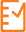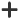Log on to rate and give feedback    1 2 3 4 5
5How to 8/1/2022
Products: AS-B, Enterprise Central, Enterprise Server, AS-P, Project Configuration Tool
Functionalities: Trends
Product version: 3.2, 3.3, 2022

# Creating a Log Processing Trend Log

You create a log processing trend log to enable calculation within or between various trend logs.HideA log processing trend log enables calculation within or between various trend logs. With this feature, building managers can gather new information about how the building is operating, they can view historical data that is calculated against new data and in this way detect new trends, they can compare various values and calculate a new trend log displaying relativised behavior, or unify measurements made with different units. The results from these calculations can indicate anomalies that have other reasons than the naturally varying conditions, so that it becomes easier to find problems with the building.

## Examples

For instance, you use a log processing trend log in the following contexts:

Use a log processing trend log to compute the amount of the energy used during some given period of time, depending on the degree of the outdoor temperature. You can log this value along with, or instead of, the value of the outdoor temperature. You can also use some other energy measure such as degree days or an energy index listed in MSCI World Index. For this calculation you use the formula expressing the following function: “Energy consumption divided by energy index"

Use a log processing trend log to compute how much the light level differs from the natural light level. For this calculation you use the formula expressing the following function: "natural light level minus light level in the room".

Use a log processing trend log to store in a new log the average, or the minimum or the maximum temperature for a given period of time. You can log this value along with, or instead of, the value of the temperature. It can be further used by a 3rd party reporting tool. For this calculation you use one of the formulas expressing one of the following functions: "average(temp)" or "min(temp)" or again "max(temp)".

Use a log processing trend log to store in a new log the value of the total energy usage. You can log this value along with, or instead of, the values of the partial energy indicators. For this calculation you use the formula expressing the following function: "a sum of energy usage measured by multiple meters where there is no main meter".

## Mathematical Operators Used in Calculations

The formulas for the calculations use operators discribed in the C++ Mathematical Expression Toolkit Library (ExprTk). For more information, see: https://github.com/ArashPartow/exprtk Scroll down to [SECTION 01 - CAPABILITIES] and [SECTION 02 - EXAMPLE EXPRESSIONS].

For instance, use:

(01) Basic operators: +, -, *, /, %, ^

(02) Assignment: :=, +=, -=, *=, /=, %=

(03) Equalities & Inequalities: =, ==, <>, !=, <, <=, >, >=

(04) Logic operators: and, mand, mor, nand, nor, not, or, shl, shr, xnor, xor, true, false

To create a log processing trend log
1. In Workstation, in the System Tree pane select the folder where you want to create the log processing trend log.

2. On the File menu, point to New, and then click Trend .

3. In the object type list, select Log Processing Trend Log .

4. In the Configure Log Processing Trend Log dialog box of the Create Object wizard, in the Name box, type the name of your log.

5. In the Path , select the folder where you want to create the log processing trend log .

6. In the Description box, type a description for the trend log.

7. Click Next .

8. In the Start time boxes, enter the starting date and the starting time of the calculation.

9. In the Period interval box, type the time span of the calculation.

10. Clickto add trend logs to be used in your calculation.

11. In the Choosing the Type and Naming the Object dialog box of the Create Object wizard, Name box, type the name of your trend log.

12. In the Path box, select the folder where you want to create the log processing trend logs.

13. In the Description box, type a description for the trend log.

14. Click Next .

15. In the Configure Log Processing Trend Log Item dialog box of the Create Object wizard, in the Log reference box, select the unique identifier of your trend log.

16. In the Name box, type the name of your trend log.

17. In the Calculation type box, select the calculation type for your trend log.

18. In the Use last know value , select True to use the last know value from the trend log.

19. In the Log unit , enter the input unit of your log.

20. In the Target unit , enter the output unit of your log.

21. In the Log guid , select the unique identifier of the log.

22. Click Create .

23. In the Configure Log Processing Trend Log dialog box of the Create Object wizard, select a trend log from the filter that you added and that are displayed in the Quick filter list.

24. In the Time zone box, select the time zone for your calculation.

25. In the Displaying timestamp select the point in calculations where you want your timestamp to be inserted.

26. In the Unit , enter the unit of your log.

27. In the Fetch delay , select the waiting time before the calculation starts.

28. In the Persist data , select True to store data in the database.

Note:

Storing the data in a database can increase performance if you have multiple Log Processing Trend Logs.

29. Click Create .

Community Content

### Language

 © 2022 Schneider Electric Terms of Service Data Privacy Change your cookie settings